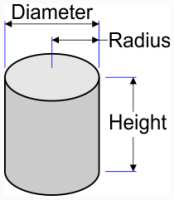# Calculating the Volume and Surface Area of a Cylinder

## The Cylinder

A cylinder is the revolution of a line segment around an axis. It’s also the translation of a disc along its own axis.

Its volume is calculated by multiplying the area of the disc by its height.

Its lateral area is calculated by multiplying the perimeter by its height.

The total surface area is calculated by adding the lateral area and the area of the two discs making up its top and bottom.

## Cylinder calculationRadius Diameter : Height: Unit: inch foot yard mile millimeter centimeter decimeter meter décameter hectometer kilometer Decimal places: 0 1 2 3 4 5 6 7 8 9 10 Perimeter: 16,96 cm Disk area: 22,90 cm2 Lateral area: 91,61 cm2 Total area: 137,41 cm2 Volume : 123,67 cm3

## The Cylinder of an Internal Combustion Engine

The surface area of a motor’s cylinder is calculated in the same manner: The stroke is the value for the height in the formula above, and the bore divided by two corresponds to the radius. Now you just need to multiply these results by the number of cylinders in the engine, and there you go!

In the following formula, the preceding numbers are used. You just need to know how many cylinders the engine has:

 Number of cylinders : Total Capacity: 742,03 cm3

## A Few Uses for Knowing a Cylinder’s Volume

Calculating a cylinder’s volume is also handy for determining the amount of water in a tube or a canal. If you know the interior diameter and the length, you can very easily solve for the volume, and therefore, the weight of the water.

For example, a hose with a diameter of 19mm and a height of 25m which was left in the sun contains 7.09 liters of warm water. Knowing this, you know that you need to remove this much water before watering your plants – if you don’t want to fry them that is!

Calculating the lateral area of a cylinder allows to you determine the area of the heat from a heat exchanger or from a coiled radiator. For example, a coil with a diameter of 8 mm and a height of 10 m has an exchange area of 0.25 m2. This can be useful when calculating the area of solar thermal panels, cooling radiators or even of the heating circuits for floors.

## Drawing a Cylinder

You can draw a cylinder in different perspectives by replacing its circles with ellipses. The larger of the ellipse’s radii corresponds to the cylinder’s radius, and its small radius is equal to the large radius multiplied by the sine of the angle in perspective.

Thus, a 45° perspective will make an ellipse whose small radius is equal to 0.707 times the large radius.

## The Volume of a Cylinder

To find the volume of a cylinder, you just need to multiply the circular base by the height. Thus, you solve the formula in two steps: one, calculating the area of the disk by multiplying the radius by itself and then by Pi (3.1415927...), and two, by multiplying the preceding result by the height.

Calculating the cylinder capacity for motors – either two-stroke or four-stroke internal combustion engines – is very similar. The difference is that one would typically use the diameter and not the radius to calculate the disk’s area. This diameter is usually called the “bore”.

So the formula becomes (D2/4)*Pi: diameter (or bore) times itself, divided by 4, and then multiplied by Pi. then, you multiply the circular area obtained by the stroke, or the height, just as before. Now you have the unit cylinder. Finally, if the engine has several cylinders, you just need to multiply the unit cylinder by the number of cylinders in order to find the total displacement, also called the total capacity.

## Other Cylinders

A roller is a cylinder (typically full, such as a steamroller).

A tube is a hollow cylinder.